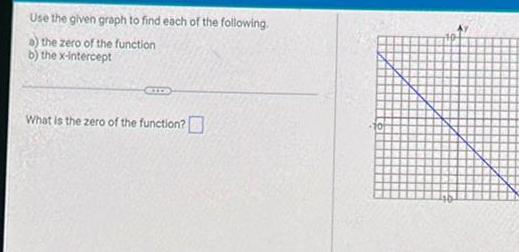Question:

# Use the given graph to find each of the following a the zero

Last updated: 9/17/2023Use the given graph to find each of the following a the zero of the function b the x intercept What is the zero of the function D